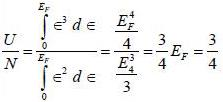Courses

# Test: Thermo And Statistical - 1

## 20 Questions MCQ Test GATE Physics Mock Test Series | Test: Thermo And Statistical - 1

Description
This mock test of Test: Thermo And Statistical - 1 for GATE helps you for every GATE entrance exam. This contains 20 Multiple Choice Questions for GATE Test: Thermo And Statistical - 1 (mcq) to study with solutions a complete question bank. The solved questions answers in this Test: Thermo And Statistical - 1 quiz give you a good mix of easy questions and tough questions. GATE students definitely take this Test: Thermo And Statistical - 1 exercise for a better result in the exam. You can find other Test: Thermo And Statistical - 1 extra questions, long questions & short questions for GATE on EduRev as well by searching above.
QUESTION: 1

### Consider a system of N non-interacting classical linear harmonic oscillates (each of mass m and angular frequency ω) in thermal equilibrium at temperature T. The enthalpy of the system is

Solution:

Partition function of the system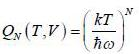Helmholtz free energy,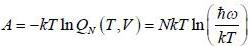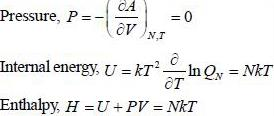QUESTION: 2

### The equation of state for adiabatic process performed on an ideal gas consisting of non-relativistic rigid triangular molecule in terms of pressure (P) and volume (V) is given by

Solution:

For rigid triangular molecule, the internal energy of a molecule,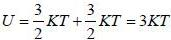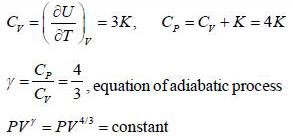QUESTION: 3

### Consider a sensitive spring balance characterized by a spring constant k0. The balance is in an environment whose temperature is T. A small object of mass m is suspended to the spring. The mean fluctuation in its position is

Solution:

Potential energy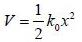Kinetic Energy K.E.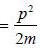By Virial theorem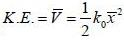By equipartition theorem,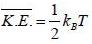Mean square fluctuation in position,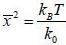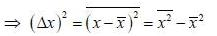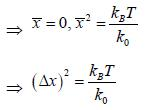QUESTION: 4

The entropy (S) of a thermodynamics system is give in terms of its internal energy (U) and volume (V) by S= C U^(3/4) V^(1/4) , where c is constant.

The pressure (P) of the system is given by

Solution: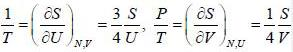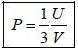QUESTION: 5

Which of the following can exhibit Bose-Einstein condensation, even in principle?

Solution:

Photon is surely boson

QUESTION: 6

Two gases are separated by an impermeable but movable and conducting partition in an isolated container. Both gases are in which type of equilibrium?

Solution:

For conducting, non-rigid and impermeable partition, temperature and pressure are constants.

QUESTION: 7

Which option is not correct for a liquid to vapour phase transition at critical point?

Solution:

Specific heat is second order derivatives of Gibb's free energy and it has finite discontinuity at transition point.

*Answer can only contain numeric values
QUESTION: 8

One mole of an ideal gas at 300K expands reversibly and isothermally from a pressure of 1.5 x 103 N/mto 5 x 102 N/m2. The change in entropy (J/K) of the universe is _____________.

Solution:

For reversible process change in entropy of universe (ΔS) = zero.

*Answer can only contain numeric values
QUESTION: 9

Consider a photon gas inside a 3-D cavity of volume V and in thermal equilibrium at temperature T. If photon gas is expanded adiabatically into vacuum to 16 times of initial volume, then its temperaure changes to n times of initial tempeature. The value of n is _________________.

Solution:

The process is free expansion For free expansion internal energy (U) = constant.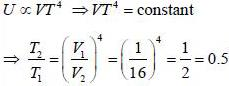*Answer can only contain numeric values
QUESTION: 10

Six distinghuishable particles are distributed over three non-degenerate levels of energies 0, ∈ and 2∈. The energy of most probable macrostate of the system is _______ ∈.

Solution:

For most probable macrostate particles are distributed equally in all groups.
Energy of the system,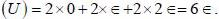*Answer can only contain numeric values
QUESTION: 11

A branch of excitations for a 3-dimension system has a dispersion relation, ∈(k) = A k2/3 where, A is constant, ∈ is energy' and k is the magnitude of wave vector. The excitations are bosonic in nature and chemical potential is zero. The entropy(S) of the system varies on absolute temperature (T) as S ∝ Tn/2, the value of n is

Solution: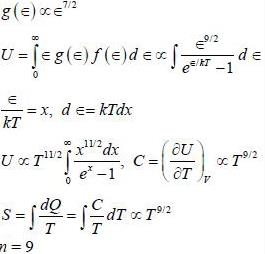*Answer can only contain numeric values
QUESTION: 12

The entropy of a system of N spins, which may align either in the upward or in the downward direction. The probability of upward directions is 1/3 and that of downward direction is 2/3. The entropy per spin in unit of kB is _______

Solution: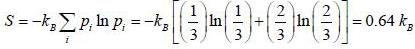*Answer can only contain numeric values
QUESTION: 13

Consider a system of two ising spin S1 and S2 taking value ±1 with interaction energy ∈ = -JS1S2 in thermal equilibrium at temperature T. The partition function of the system in the limit T → ∞ is ______.

Solution: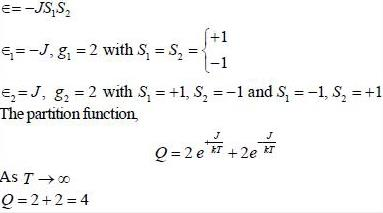QUESTION: 14

Consider a system that has two energy' levels of energy 0 and ∈. The lowest level is doubly degenerate while excited level is triply degenerate. The system is in thermal equilibrium at temperature T. The average energy of the system in very high temperature limit is

Solution:

The average energy of the system,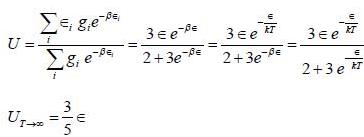QUESTION: 15

What is the maximum entropy of the system when six distinguishable particles are distributed over three non-degenerate energy states of energies 0, ∈ and 2 ∈ ?

Solution:

For maximum entropy particles are distributed equally.
The number of microstates for equal distribution.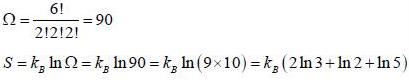QUESTION: 16

The Helmholtz free energy (A) of atherm odynam ic system in terms of volum e (V) and temperature (T) is given by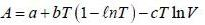where a. b and c are constants The enthalpy (H) of the system is

Solution: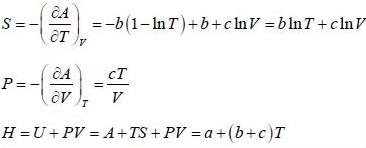QUESTION: 17

One mole of an ideal gas at 27°C expands reversibly and isothermally from a pressure of 2 x 106 N/m2 to a pressure 2 x 105 N/m2 The change in entropy (in J/K) of the gas is

Solution: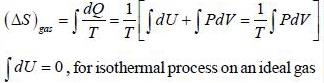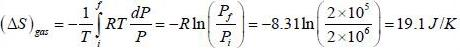QUESTION: 18

A process is performed on 2 moles of an ideal mono-atomic gas in which its entropy depends an absolute temperature T as S = α/T, where α is a constant. What is the work performed by the eas if its temperature varies from T1 to T2?

Solution:

By using combined fonn of first and second law of thermodynamics
dW = TdS - dU
Work performed by the gas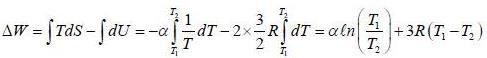QUESTION: 19

For a system of non-interacting one-dimension quantum oscillators of angular frequency ω, the value of enthalpy in ther limit per oscillator T → 0, is

Solution:

Tlie partition function for a quantum oscillator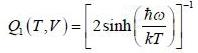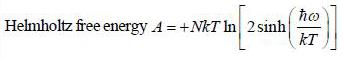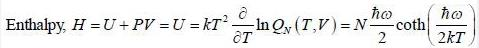In the limit, T → 0 , enthalpyper oscillator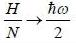QUESTION: 20

Consider an ideal gas of NFemnons inside a 3-D container of volume V. Assume that the dispersion relation ∈ (k) x ∝, the ratio of the ground state energy per particle to the Fermi energy at absolute zero of temperature is

Solution: